# Circle - simple

Calculate the area of a circle in dm2 if its circumference is 31.4 cm.

S =  0.78 dm2

### Step-by-step explanation: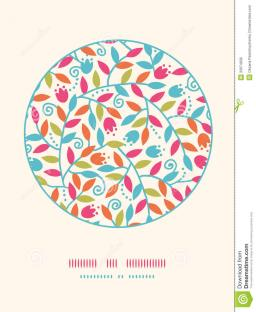Did you find an error or inaccuracy? Feel free to write us. Thank you!#### You need to know the following knowledge to solve this word math problem:

We encourage you to watch this tutorial video on this math problem:

## Related math problems and questions:

• Area to perimeterCalculate circle circumference if its area is 254.34cm2
• Four circles1) Calculate the circle radius if its area is 400 cm square 2) Calculate the radius of the circle whose circumference is 400 cm. 3) Calculate circle circumference if its area is 400 cm square 4) Calculate the circle's area if perimeter 400 cm.
• Quarter of a circleCalculate the circumference of a quarter circle if its content is S = 314 cm2.
• Area of a circle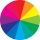The circumference of the circle is 150.72 centimeters. Calculate the area of the circle.
• Math heart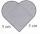Stylized heart shape created from a square with side 5 cm and two semicircles over his sides. Calculate the content area and its circumference.
• Circle simpleCircumference of a circle is 6.28. What is the area of the circle?
• Perimeter of circleCalculate the circumference of described circle to the triangle with sides 9,12,15 cm.
• Perimeter of rhombus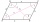The area of the rhombus is 32 cm2. One side of it measures 10 cm. The height on the other side measures 5 cm. What is the circumference of this rhombus?
• Round skirt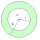The cut on the round skirt has the shape of an annulus. Determine how much m² of fabric will be consumed on an 80 cm long skirt. The circumference of the waist is a circle with a smaller radius and is 69 cm.
• CircleHow big is an area of a circle if its circumference is 51.2 cm?
• Ratio of sidesCalculate the area of a circle with the same circumference as the circumference of the rectangle inscribed with a circle with a radius of r 9 cm so that its sides are in ratio 2 to 7.
• Silo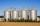Outer perimeter of silo is 32 m. Concrete wall is 35 cm thick. What is diameter and area of inside floor of silo?
• Rhombus 4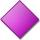The circumference of the rhombus is 44 cm, its height is 89 mm long. Calculate its content area.
• Isosceles trapezoidCalculate the circumference and the contents of the isosceles trapezoid if you know the size of the bases is 8 and 12 cm and the size of the arms is 5 cm.
• Circle - easy 2The circle has a radius 6 cm. Calculate:
• Circle and hexagon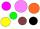Calculate the radius of a circle whose circumference is 8.4 cm longer than the inscribed regular hexagon's circumference.
• SquareCalculate the perimeter and the area of square with a diagonal length 30 cm.